# RD Sharma Solutions - Chapter 18 - Practical Geometry (Constructions) (Part - 1), Class 8, Maths Notes | Study RD Sharma Solutions for Class 8 Mathematics - Class 8

## Class 8: RD Sharma Solutions - Chapter 18 - Practical Geometry (Constructions) (Part - 1), Class 8, Maths Notes | Study RD Sharma Solutions for Class 8 Mathematics - Class 8

The document RD Sharma Solutions - Chapter 18 - Practical Geometry (Constructions) (Part - 1), Class 8, Maths Notes | Study RD Sharma Solutions for Class 8 Mathematics - Class 8 is a part of the Class 8 Course RD Sharma Solutions for Class 8 Mathematics.
All you need of Class 8 at this link: Class 8

PAGE NO 18.10:

Question 1:

Construct a quadrilateral ABCD, in which AB  = 6 cm, BC  = 4 cm, CD  = 4 cm, ∠B  = 95° and ∠C  = 90°.

Steps of construction:

Step I: Draw BC = 4 cm.

Step II: Construct ∠ABC = 95° at B.

Step III : With B as the centre and radius 6 cm, cut off BA = 6 cm.

Step IV: Construct ∠BCD = 90° at C.

Step V :With C as the centre and radius 4 cm, cut off BA = 4 cm.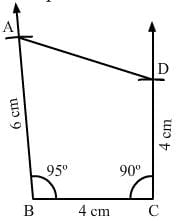Question 2:

Construct a quadrilateral ABCD, where AB  = 4.2 cm, BC  = 3.6 cm, CD  = 4.8 cm, ∠B  = 30° and ∠C  = 150°.

Steps of construction:

Step I: Draw BC = 3.6 cm.

Step II: Construct ∠ABC = 30° at B.

Step III : With B as the centre and radius 4.2 cm, cut off BA = 4.2 cm.

Step IV: Construct ∠BCD = 150° at C.

Step V :With C as the centre and radius 4.8 cm, cut off CD = 4.8 cm.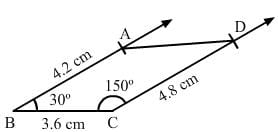Question 3:

Construct a quadrilateral PQRS, in which PQ  = 3.5 cm, QR  = 2.5 cm, RS  = 4.1 cm, ∠Q  = 75° and ∠R  = 120°.

Steps of construction:

Step I: Draw QR = 2.5 cm.

Step II: Construct ∠PQR = 75° at Q.

Step III : With Q as the centre and radius 3.5 cm, cut off QP = 3.5 cm.

Step IV: Construct ∠QRS = 120° at  R.

Step V :With R as the centre and radius 4.1 cm, cut off RS = 4.1 cm.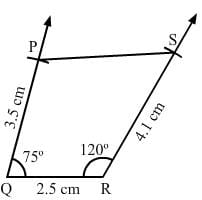PAGE NO 18.11:

Question 4:

Construct a quadrilateral ABCD given BC  = 6.6 cm, CD  = 4.4 cm, AD  = 5.6 cm and ∠D  = 100° and ∠C  = 95°.

Steps of construction:

Step I: Draw DC = 4.4 cm.

Step II: Construct ∠ADC = 100° at D.

Step III : With D as the centre and radius 5.6 cm, cut off DA = 5.6 cm.

Step IV: Construct ∠BCD = 95° at C.

Step V :With C as the centre and radius 6.6 cm, cut off CB = 6.6 cm.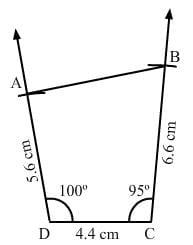Question 5:

Construct a quadrilateral ABCD, in which AD  = 3.5 cm, AB  = 4.4 cm, BC  = 4.7 cm, ∠A  = 125° and ∠B  = 120°.

Steps of construction:

Step I: Draw AB = 4.4 cm.

Step II: Construct ∠BAD = 125° at A.

Step III : With A as the centre and radius 3.5 cm, cut off  AD = 3.5 cm.

Step IV: Construct ∠ABC = 120° at B.

Step V :With B as the centre and radius 4.7 cm, cut off  BC = 4.7 cm.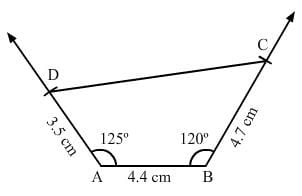Question 6:

Construct a quadrilateral PQRS, in which ∠Q = 45°, ∠R  = 90°, QR  = 5 cm, PQ  = 9 cm and Rs = 7 cm.

Steps of construction:

Step I: Draw QR = 5 cm.

Step II: Construct ∠PQR = 45° at Q.

Step III : With Q as the centre and radius 9 cm, cut off QP = 9 cm.

Step IV: Construct ∠QRS = 90° at R.

Step V :With R as the centre and radius 7 cm, cut off RS = 7 cm.

Since, the line segment PQ and RS intersect each other, the quadrilateral cannot be constructed.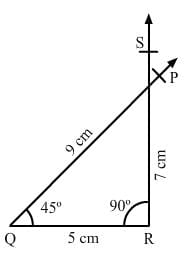Question 7:

Construct a quadrilateral ABCD, in which AB  =  BC  = 3 cm, AD  = 5 cm, ∠A  = 90° and ∠B  = 105°.

Steps of construction:Step I: Draw AB = 3 cm.

Step II: Construct ∠DAB = 90° at A.

Step III : With A as the centre and radius 5 cm, cut off  AD = 5 cm.

Step IV: Construct ∠ABC = 105° at B.

Step V: With B as the centre and radius 3 cm, cut off BC = 3 cm.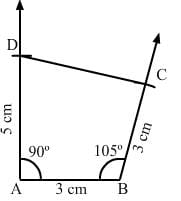Question 8:

Construct a quadrilateral BDEF, where DE  = 4.5 cm, EF  = 3.5 cm, FB  = 6.5 cm, ∠F  = 50° and ∠E  = 100°.

Steps of construction:

Step I: Draw EF = 3.5 cm.

Step II: Construct ∠DEF = 100° at E.

Step III : With E as the centre and radius 4.5 cm, cut off  DE = 4.5 cm.

Step IV: Construct ∠EFB = 50° at F.

Step V :With F as the centre and radius 6.5 cm, cut off FB = 6.5 cm.

Step VI: Join BD. The quadrilateral so obtained is the required quadrilateral.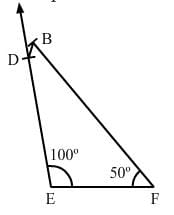The document RD Sharma Solutions - Chapter 18 - Practical Geometry (Constructions) (Part - 1), Class 8, Maths Notes | Study RD Sharma Solutions for Class 8 Mathematics - Class 8 is a part of the Class 8 Course RD Sharma Solutions for Class 8 Mathematics.
All you need of Class 8 at this link: Class 8Use Code STAYHOME200 and get INR 200 additional OFF

## RD Sharma Solutions for Class 8 Mathematics

88 docs

Track your progress, build streaks, highlight & save important lessons and more!

,

,

,

,

,

,

,

,

,

,

,

,

,

,

,

,

,

,

,

,

,

,

,

,

,

,

,

;